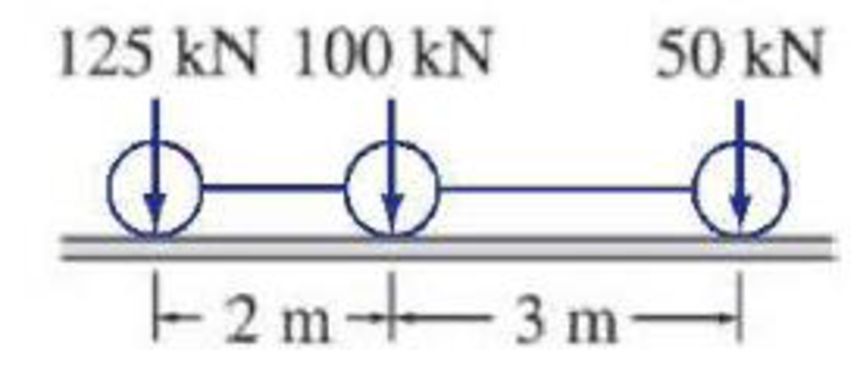# Determine the absolute maximum shear in a 15 m long simply supported beam due to the series of three moving concentrated loads shown in Fig. P9.13. FIG. P9.13, P9.17, P9.18, P9.22

#### Solutions

Chapter
Section
Chapter 9, Problem 18P
Textbook Problem
52 views

## Determine the absolute maximum shear in a 15 m long simply supported beam due to the series of three moving concentrated loads shown in Fig. P9.13.FIG. P9.13, P9.17, P9.18, P9.22

To determine

Find the absolute maximum shear in a 15 m long simply supported beam.

### Explanation of Solution

Calculation:

Sketch the simply supported beam as shown in Figure 1.

The simply supported beam the maximum shear occurs at support. Hence, find the influence line diagram for support reactions.

Case 1: Consider the Absolute Maximum Shear occurs at Support A.

Influence line diagram for support reaction at A.

Apply a 1 k unit moving load at a distance of x from left end A.

Sketch the free body diagram of beam as shown in Figure 1.

Refer Figure 1.

Find the equation of support reaction (Ay) at A using equilibrium equation:

Consider moment equilibrium at point B.

Consider clockwise moment as positive and anticlockwise moment as negative

Sum of moment at point B is zero.

ΣMB=0Ay(15)1(15x)=0Ay(15)15+x=015Ay=15x

Ay=1x15        (1)

Find the equation of support reaction (By) at B using equilibrium equation:

Apply vertical equilibrium equation of forces.

Consider upward force as positive (+) and downward force as negative ().

Ay+By=1

Substitute 1x30 for Ay.

1x15+By=1By=11+x15By=x15        (2)

Consider Equation (1).

Find the value of influence line ordinate of reaction Ay at support A.

Substitute 0 for x in Equation (1).

Ay=1015=1kN

Similarly calculate the influence line ordinate of reaction Ay for different value of x and summarize the result in Table 1.

 x (m) Ay (kN/kN) 0 1 15 0

Draw the influence line diagram for the vertical reactions at support A using Table 1 as shown in Figure 3.

Refer Figure 3.

Find the slope (θAB) of influence line diagram of Ay.

θAB=1L

Here, L is the length of the beam.

Substitute 15 m for L.

θAB=115

Find the maximum absolute maximum shear for case 1.

Sketch the loading position on the beam when the load 1 placed at just right of A as shown in Figure 5.

Refer Figure 5.

Find the shear force of case 1.

SA=[125(L)+100(L2)+50(L5)](θAB)

Substitute 15 m for L and 115 for θAB

### Still sussing out bartleby?

Check out a sample textbook solution.

See a sample solution

#### The Solution to Your Study Problems

Bartleby provides explanations to thousands of textbook problems written by our experts, many with advanced degrees!

Get Started

Find more solutions based on key concepts
What is the most common type of chart in engineering?

Engineering Fundamentals: An Introduction to Engineering (MindTap Course List)

What are two reasons why a hole may need to be countersunk?

Precision Machining Technology (MindTap Course List)

What is a common problem in calculating start and finish times? Provide an example.

Systems Analysis and Design (Shelly Cashman Series) (MindTap Course List)

Describe the uses of tags. List steps to tag digital content.

Enhanced Discovering Computers 2017 (Shelly Cashman Series) (MindTap Course List)

What is a resource? What are the two types?

Principles of Information Security (MindTap Course List)

What two welding processes are semiautomatic processes?

Welding: Principles and Applications (MindTap Course List)

If your motherboard supports ECC DDR3 memory, can you substitute non-ECC DDR3 memory?

A+ Guide to Hardware (Standalone Book) (MindTap Course List)